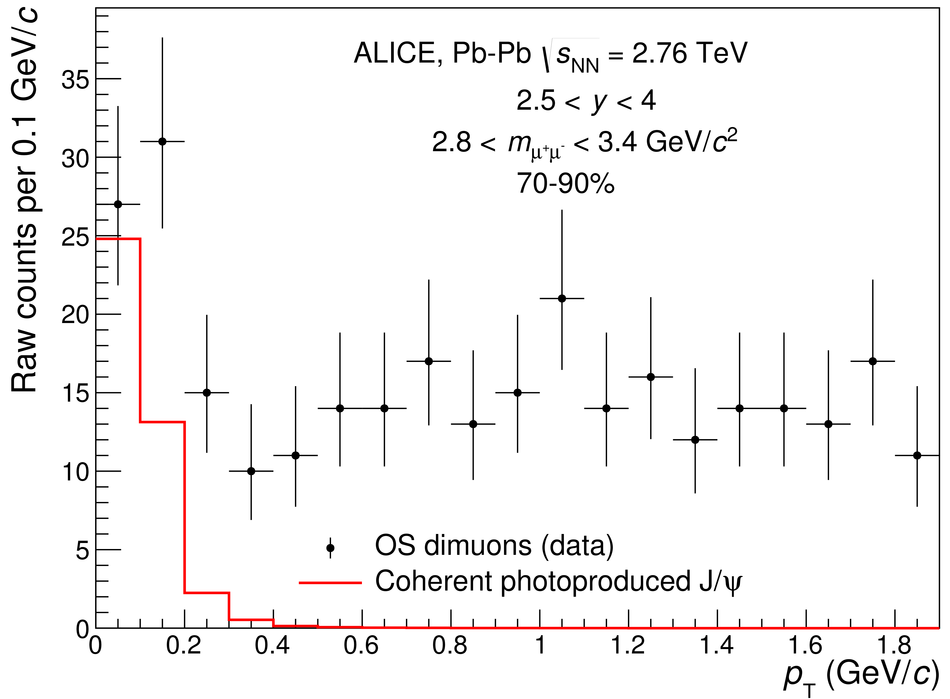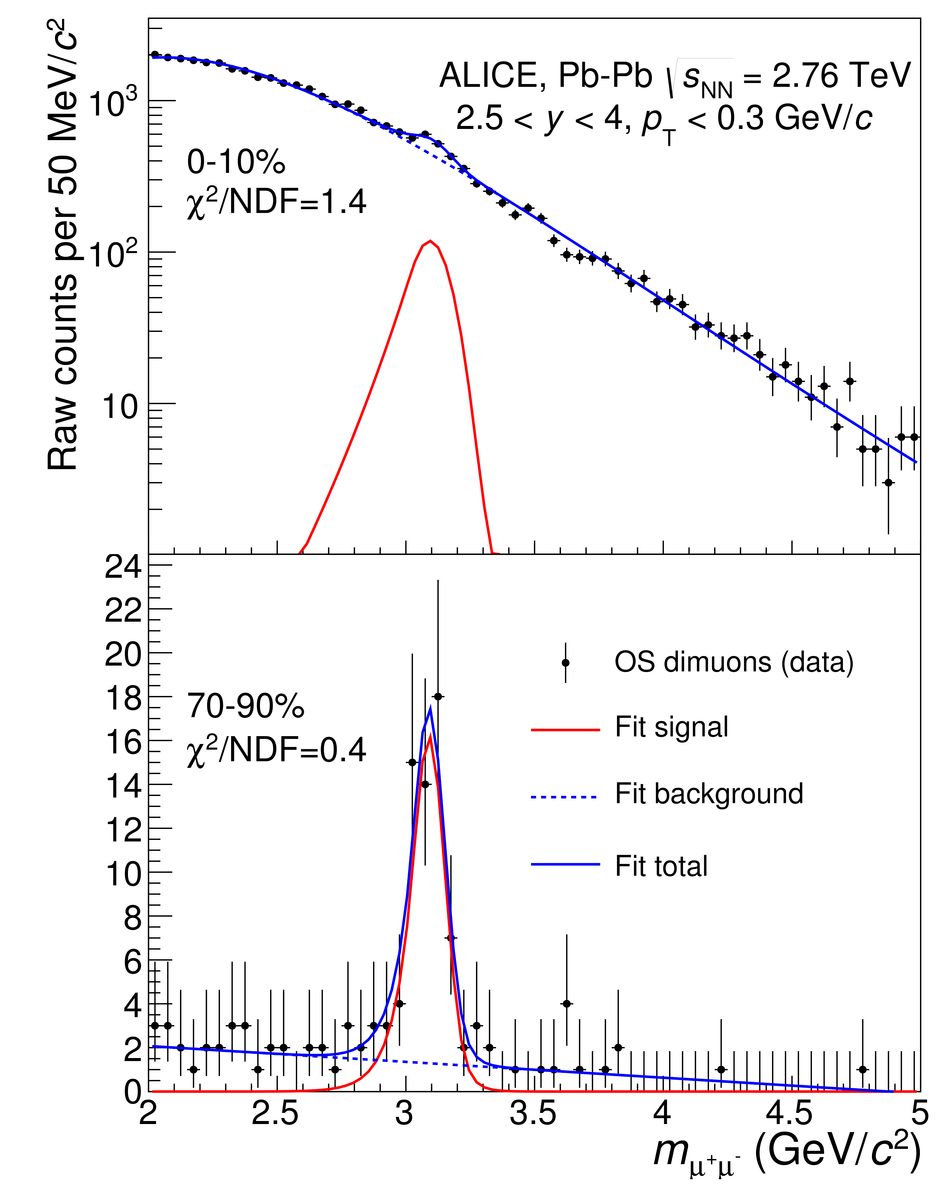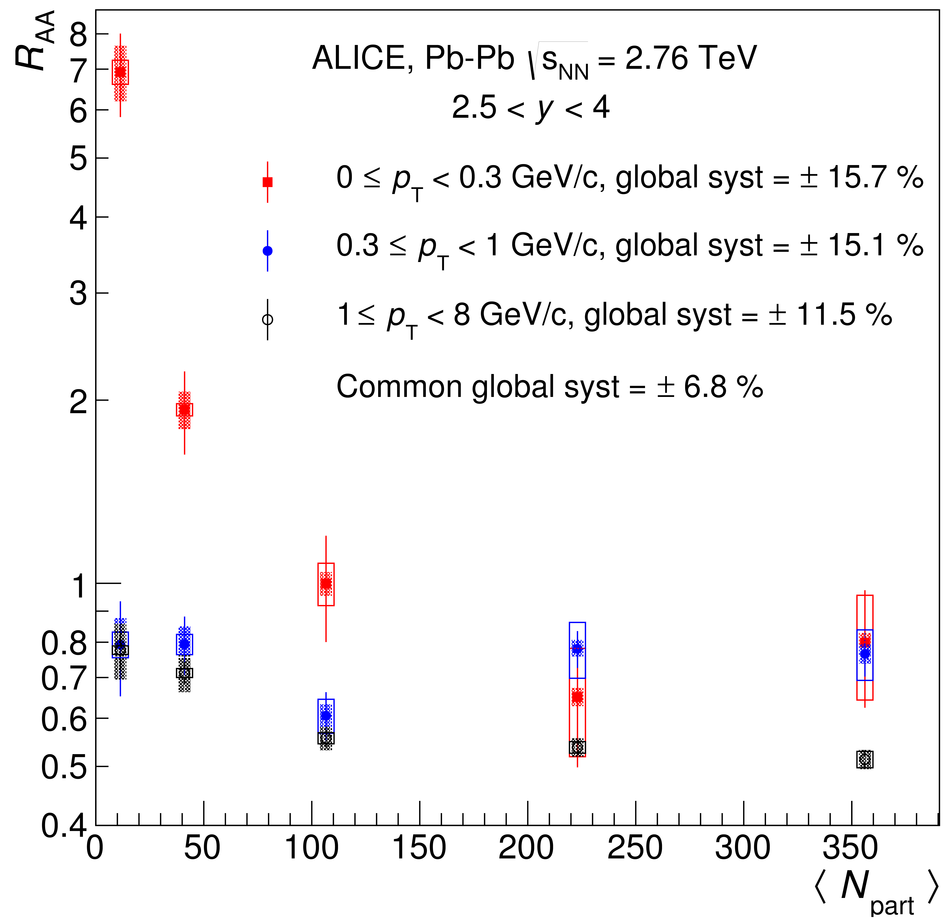# Measurement of an excess in the yield of J/$ψ$ at very low $p_{\rm T}$ in Pb-Pb collisions at $\sqrt{s_{\rm NN}}$ = 2.76 TeV

We report on the first measurement of an excess in the yield of J/$\psi$ at very low transverse momentum ($p_{\rm T}<~ 0.3$ GeV/$c$) in peripheral hadronic Pb-Pb collisions at $\sqrt{s_{\rm NN}}$ = 2.76 TeV, performed by ALICE at the CERN LHC. Remarkably, the measured nuclear modification factor of J/$\psi$ in the rapidity range $2.5<~y<~4$ reaches about 7 (2) in the $p_{\rm T}$ range 0-0.3 GeV/$c$ in the 70-90% (50-70%) centrality class. The J/$\psi$ production cross section associated with the observed excess is obtained under the hypothesis that coherent photoproduction of J/$\psi$ is the underlying physics mechanism. If confirmed, the observation of J/$\psi$ coherent photoproduction in Pb-Pb collisions at impact parameters smaller than twice the nuclear radius opens new theoretical and experimental challenges and opportunities. In particular, coherent photoproduction accompanying hadronic collisions may provide insight into the dynamics of photoproduction and nuclear reactions, as well as become a novel probe of the Quark-Gluon Plasma.

Figures

## Figure 1

 Raw OS dimuon $p_{\mathrm T}$ distribution for the invariant mass range 2.8 $< m_{\mu^+\mu^-}<$ 3.4 Gev/$c^2$ and centrality class 70-90$\%$. Vertical error bars are the statistical uncertainties. The red line represents the $p_{\mathrm T}$ distribution of coherently photoproduced J/$\psi$ as predicted by the STARLIGHT MC generator in Pb-Pb ultra-peripheral collisions and convoluted with the response function of the muon spectrometer. The normalization of the red line is given by the measured number of J/$\psi$ in excess reported in Table  after correction for the $\psi$(2S) feed-down and incoherent contributions (see text).## Figure 2

 Invariant mass distributions of OS dimuons in the $p_{\mathrm T}$ range 0-0.3 $\gevc$. The centrality classes are 0-10$\%$ (top) and 70-90$\%$ (bottom). Vertical error bars are the statistical uncertainties.## Figure 3

 J/$\psi$ $\raa$ as a function of $\langle N_\mathrm{part} \rangle$ for 3 $p_{\mathrm T}$ ranges in Pb-Pb collisions at $\sqrt{s_{\rm NN}}$ = 2.76 TeV. See text for details on uncertainties. When assuming full transverse polarization of the J/$\psi$ in Pb-Pb collisions, as expected if J/$\psi$ are coherently photoproduced, the $\raa$ values increase by about 21$\%$ in the range 0 $< p_{\mathrm T} <$ 0.3 GeV/$c$.